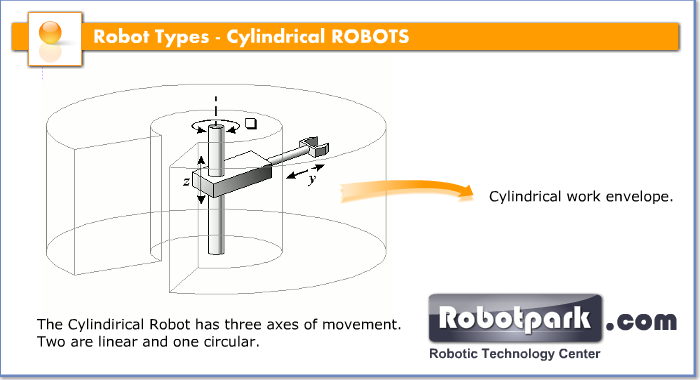## CYLINDIRICAL ROBOTS

Cylindrical Coordinate Robots are robots whose axes form a cylindrical coordinate system. Used for

-assembly operations,
-handling at machine tools,
-spot welding, and
-handling at die casting machines.

The motion of the main arm is up and down. The robot can perform this motion by extending a cylinder that’s built into the arm. In most cylindrical robots, the up-and-down motion is provided by a pneumatic cylinder, and the rotation is generally provided by a motor and gears.Cylindrical Coordinate System

A cylindrical coordinate system is a three-dimensional coordinate system that specifies point positions by the distance from a chosen reference axis, the direction from the axis relative to a chosen reference direction, and the distance from a chosen reference plane perpendicular to the axis. The latter distance is given as a positive or negative number depending on which side of the reference plane faces the point.

The origin of the system is the point where all three coordinates can be given as zero. This is the intersection between the reference plane and the axis.

The axis is variously called the cylindrical or longitudinal axis, to differentiate it from the polar axis, which is the ray that lies in the reference plane, starting at the origin and pointing in the reference direction.

The distance from the axis may be called the radial distance or radius, while the angular coordinate is sometimes referred to as the angular position or as the azimuth. The radius and the azimuth are together called the polar coordinates, as they correspond to a two-dimensional polar coordinate system in the plane through the point, parallel to the reference plane. The third coordinate may be called the height or altitude (if the reference plane is considered horizontal), longitudinal position,or axial position.

Cylindrical coordinates are useful in connection with objects and phenomena that have some rotational symmetry about the longitudinal axis, such as water flow in a straight pipe with round cross-section, heat distribution in a metal cylinder, electromagnetic fields produced by an electric current in a long, straight wire, and so on.

It is sometimes called “cylindrical polar coordinate”and “polar cylindrical coordinate”, and is sometimes used to specify the position of stars in a galaxy (“galactocentric cylindrical polar coordinate”).